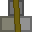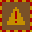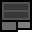# Account 483744

## BalanceMetal: 8s11 = 139Explosives: 20s6 = 326RC Turrets: 0

## Transfer

 PIN Receiver ReferenceMetal 16 × +Explosives 16 × +RC Turrets

## Create new account token

PIN:

New accounts cost 2 stacks (32 units) metal. You must give the token directly to the other player. Do not create the account yourself and give them the details. If you are found to do this, your account will be closed and all your items taken. You are responsible for ensuring the player you provide a new account token to knows how to use dredbank properly. If many players are found who don't know how to use dredbank properly, and they all got their accounts from one player, there may be a punishment against the player who provided their accounts.

## Transactions

Type Counterparty Account Reference NumberMetalExplosivesRC Turrets
Sent 321000 0 2s0 = 32 0s0 = 0 0
Deposit 0 10s0 = 160 0s0 = 0 0
Sent 321000 0 2s0 = 32 0s0 = 0 0
Sent 321000 0 2s0 = 32 0s0 = 0 0
Sent 917024 9000 75s0 = 1200 0s0 = 0 0
Deposit 0 0s0 = 0 20s0 = 320 0
Sent 298456 1000 3s0 = 48 3s0 = 48 0
Received 852133 8888 40s0 = 640 0s0 = 0 0
Sent 852133 2000 40s0 = 640 0s0 = 0 0
Sent 852133 12345 40s0 = 640 0s0 = 0 0
Sent 789542 0 9s0 = 144 5s0 = 80 0
Sent 789542 1000 9s0 = 144 4s0 = 64 0
Sent 789542 1000 4s0 = 64 2s0 = 32 0
Sent 396705 1000 0s0 = 0 15s0 = 240 0
Sent 396705 1000 0s0 = 0 10s0 = 160 0
Deposit 0 5s0 = 80 0s0 = 0 0
Received 396705 1111 79s12 = 1276 30s6 = 486 0
Received 347112 1324 29s0 = 464 0s0 = 0 0
Deposit 1234 12s0 = 192 0s0 = 0 0
Deposit 0 0s0 = 0 6s0 = 96 0
Deposit 0 18s0 = 288 3s0 = 48 0
Sent 653090 0 0s1 = 1 0s0 = 0 0
Deposit 0 1s0 = 16 0s0 = 0 0